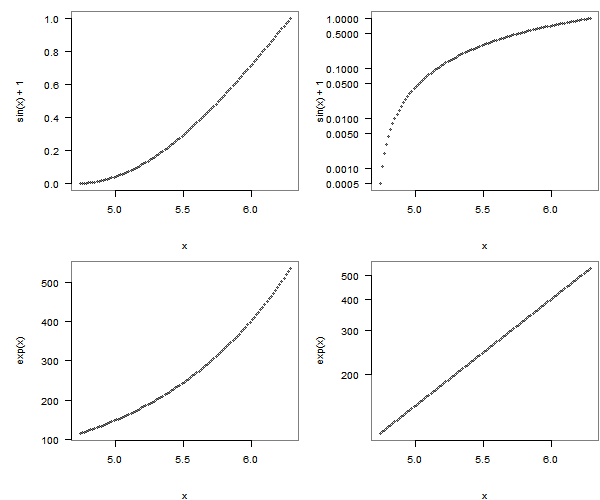# 几何级数增长应该怎样用图形表达

### 谢益辉 / 2008-10-29``````# png("exp_growth.png", width = 600, height = 500)
options(scipen = 5)
x = seq(1.51 * pi, 2 * pi, length = 100)
par(mfrow = c(2, 2), pch = 20, mar = c(5, 6, 1, 0.1),
col = rgb(0, 0, 0, 0.5), las = 1, mgp = c(4, 1, 0))
plot(x, sin(x) + 1)
plot(x, sin(x) + 1, log = "y")
plot(x, exp(x))
plot(x, exp(x), log = "y")
# dev.off()
``````